### 2.3.1.1 Nonlinear dynamics2.2

The Landau-Lifshitz-Gilbert equation is nonlinear. Therefore, the magnetic systems will present phenomena associated with nonlinearity such as stochastic resonance, nonlinear bifurcations or chaos [Fernández 00]. Furthermore, it is not possible to find exact analytical solutions except in very simple cases. The dissipation term is explicitly nonlinear, but even in the absence of this term the dynamics are nonlinear, because the effective field is dependent on the magnetization value.

To illustrate we can examine the case of a single domain particle with uniaxial anisotropy and easy axis in the Z direction under constant applied field. The energy of such particle is:(2.43)

where the first term is uniaxial anisotropy and the external field is measured in units of. We can choosewithout loss of generality and the applied field becomeswhere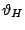is the field angle with the axis Z. The energy minimum makes an angle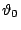with the Z axis. We can change from the laboratory reference frameto a reference framewhere the minimum position always corresponds to the Z' axis. The transformation is a rotation of anglearound the Y axis. In the small amplitude approximation, where the components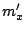and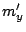of the magnetization vector are supposed to be small in comparison to component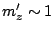, the equation of motion in the new frame of referenceis:(2.44)(2.45)

and the variables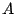,,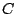and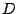are determined by equations: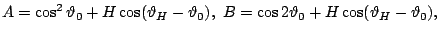(2.46)(2.47)

In the frictionless case, the solution up to second harmonic of Eq. (2.44) iswhere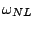is the nonlinear instantaneous frequency,is the amplitude of motion,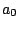is the zero harmonic and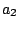is the second harmonic. The zero harmonic, which value can be obtained from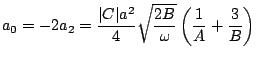(2.48)

describes the displacement of the center of the magnetization vector rotation due to the nonlinear effect and is always positive.

In the considered case of constant applied field,, we arrive to an expression for the instantaneous frequency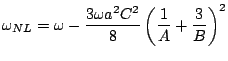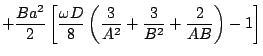(2.49)

where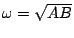. The comparison of Eq. (2.49) with numerical simulations shows good agreement for small values of the amplitude of the precessional cycle(see Fig. 2.10). This can be expected since our approximation is only valid for small amplitudes. In the case of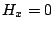we have an axially symmetric case and Eq. (2.49) reduces to.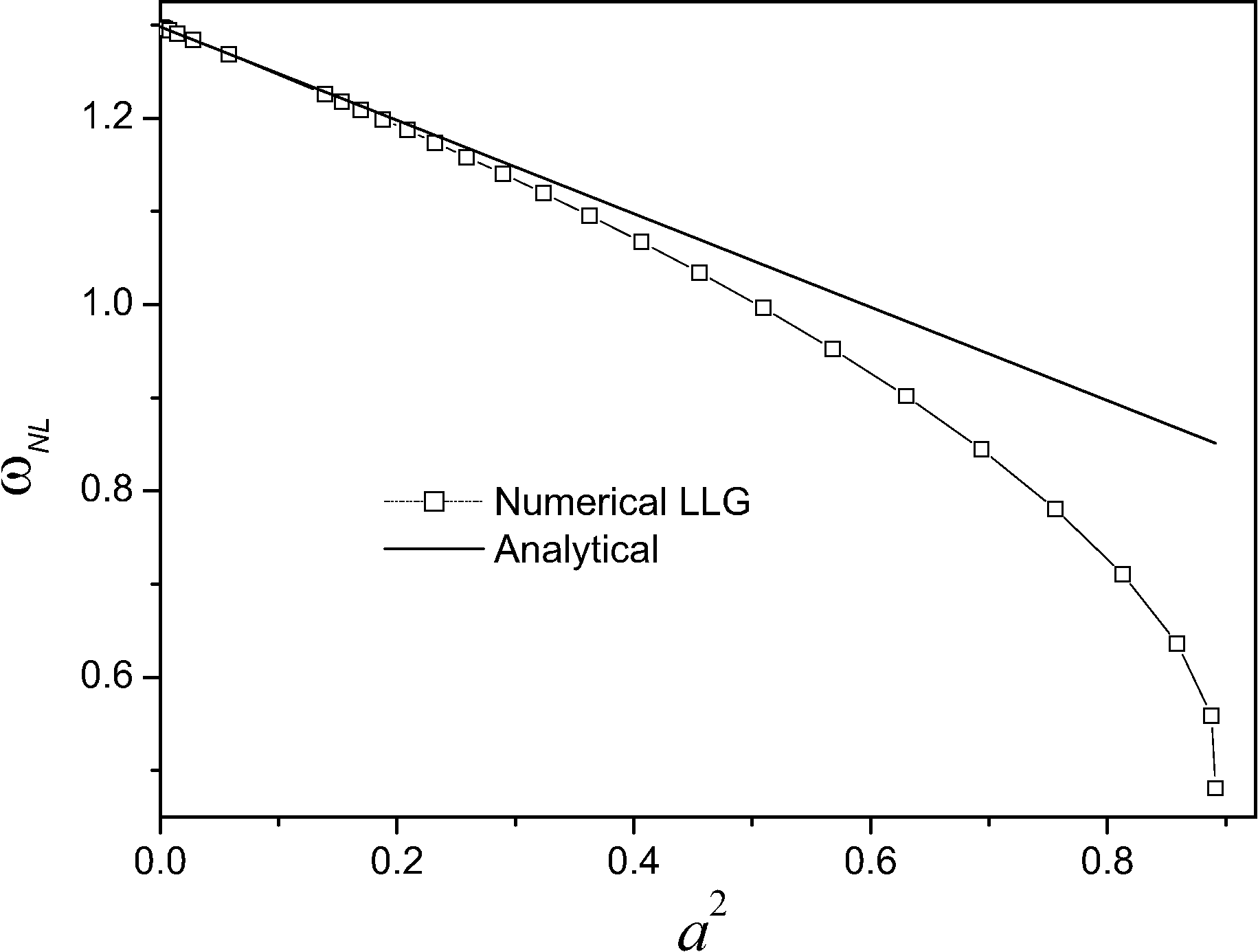The applicability of the solution can be extended including additional terms. The existence of corrections to the orbit center allows us to conclude that under numerical simulation of the LLG equation the precessional terms should not be neglected at long simulation times in the micromagnetic simulations.

2008-04-04